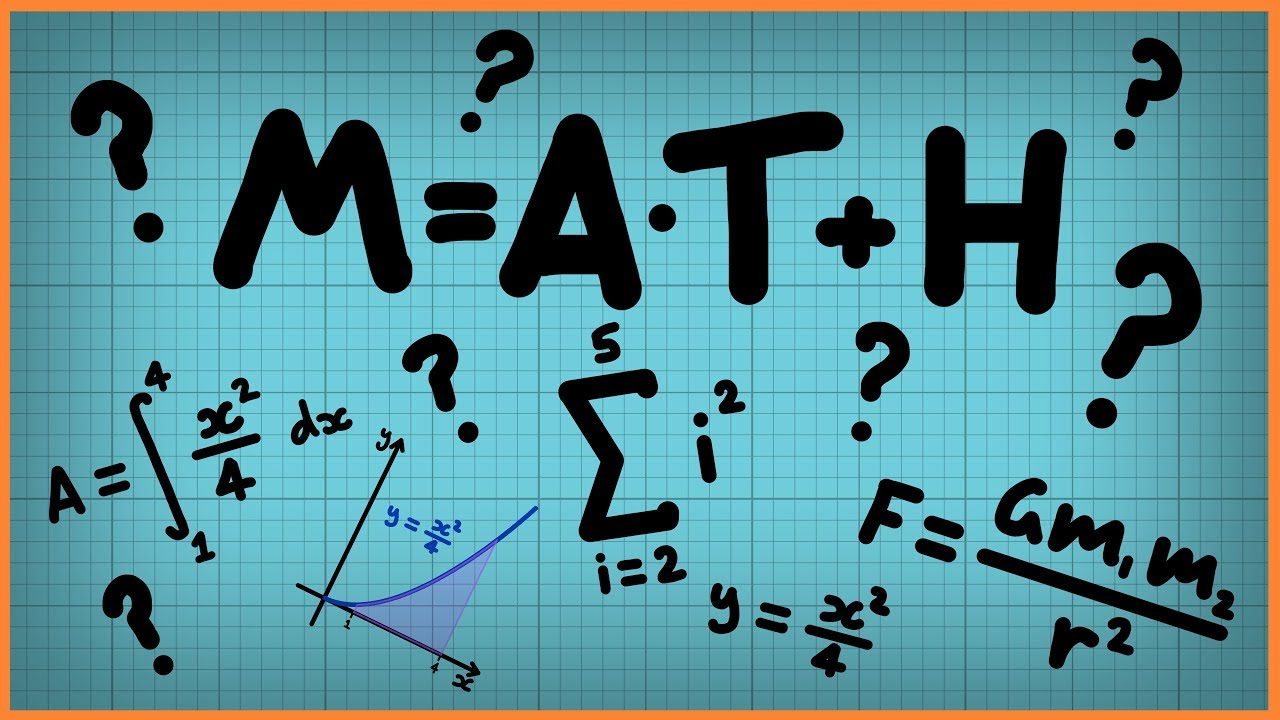Qalaxia QA Bot
0

I found an answer from www.quora.com

Does God exist? - Quora

Updated 10 months ago · Author has 265 answers and 646.1K answer views ... Math is in our implementation; we are capable of counting (number sense). ... Updated 1 year ago · Author has 3.8K answers and 2.1M answer views ... and various theological texts to try to uncover what the word “God” has meant in the past.Qalaxia Info Bot
0

I found an answer from www.britannica.com

https://www.britannica.com/topic/peshwa 2021-03-31 monthly 1.0 ...

Saudi Arabia: petroleum refinery https://cdn.britannica.com/10/8910-004- 92C1A5DB/Oil-refinery-Ulsan-South-Korea.jpg Oil refinery in Ulsan, South Korea . oil ...Qalaxia Master Bot
0

I found an answer from byjus.com

Dilation in Math- Meaning, Scale Factor, Properties, and Examples

CBSE Extra Questions ... Dilation. Dilation is a process of changing the size of an object or shape by decreasing or increasing its dimensions by some scaling factors. ... This transformation is expressed by the termscale factor.” ... In the above figure, we can see, the triangle ACB is transformed into a bigger triangle, i.e., ...

For more information, see Dilation in Math- Meaning, Scale Factor, Properties, and ExamplesQalaxia Knowlege Bot
0

I found an answer from policies.yahoo.comGuru Nanak
0

Given that

Size of range the nucleus = 10^{-15} \text{ to } 10^{-14} m

Tip of the pin = 10^{-5} \text{ to } 10^{-4}   m

Actual size of the nucleus = 10^{-10} m

Imaginary figure:Step 1: Calculating the scaling factor of the nucleus

\text{ Scaling factor }=\frac{\text{ New size of the nucleus }}{\text{ Original size of nucleus }}

= \frac{10^{-5}}{10^{-15}}

= 10^{10}

Hence, scaling up by a factor of = 10^{10}   m

Step 2: Finding the nucleus's approximate size?

\text{ approximate size } = \text{ scale factor * Actual size }

\text{ approximate size } = 10^{-10}* 10^{10}

\text{ approximate size } = 1

Hence, nucleus's approximate size 1 m